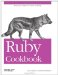# Iterating Over a Hash

Problem

You want to iterate over a hash's key-value pairs as though it were an array.

Solution

Most likely, the iterator you want is Hash#each_pair or Hash#each. These methods yield every key-value pair in the hash:

```	hash = { 1 => 'one', [1,2] => 'two', 'three' => 'three' }

hash.each_pair { |key, value| puts "#{key.inspect} maps to #{value}"}
# [1, 2] maps to two
# "three" maps to three
# 1 maps to one
```

Note that each and each_pair return the key-value pairs in an apparently random order.

Discussion

Hash#each_pair and Hash#each let you iterate over a hash as though it were an array full of key-value pairs. Hash#each_pair is more commonly used and slightly more efficient, but Hash#each is more array-like. Hash also provides several other iteration methods that can be more efficient than each.

Use Hash#each_key if you only need the keys of a hash. In this example, a list has been stored as a hash to allow for quick lookups (this is how the Set class works). The values are irrelevant, but each_key can be used to iterate over the keys:

```	active_toggles = { 'super' => true, 'meta' => true, 'hyper' => true }
active_toggles.each_key { |active| puts active }
# hyper
# meta
# super
```

Use Hash#each_value if you only need the values of a hash. In this example, each_value is used to summarize the results of a survey. Here it's the keys that are irrelevant:

```	favorite_colors = { 'Alice' => :red, 'Bob' => :violet, 'Mallory' => :blue,
'Carol' => :blue, 'Dave' => :violet }

summary = Hash.new 0
favorite_colors.each_value { |x| summary[x] += 1 }
summary
# => {:red=>1, :violet=>2, :blue=>2}
```

Don't iterate over Hash#each_value looking for a particular value: it's simpler and faster to use has_value? instead.

```	hash = {}
1.upto(10) { |x| hash[x] = x * x }
hash.has_value? 49 # => true
hash.has_value? 81 # => true
hash.has_value? 50 # => false
```

Removing unprocessed elements from a hash during an iteration prevents those items from being part of the iteration. However, adding elements to a hash during an iteration will not make them part of the iteration.

Don't modify the keyset of a hash during an iteration, or you'll get undefined results and possibly a RuntimeError:

```	1.upto(100) { |x| hash[x] = true }
hash.keys { |k| hash[k * 2] = true }
# RuntimeError: hash modified during iteration
```

Using an array as intermediary

An alternative to using the hash iterators is to get an array of the keys, values, or key-value pairs in the hash, and then work on the array. You can do this with the keys, values, and to_a methods, respectively:

```	hash = {1 => 2, 2 => 2, 3 => 10}
hash.keys # => [1, 2, 3]
hash.values # => [2, 2, 10]
hash.to_a # => [[1, 2], [2, 2], [3, 10]]
```

The most common use of keys and values is to iterate over a hash in a specific order. All of Hash's iterators return items in a seemingly random order. If you want to iterate over a hash in a certain order, the best strategy is usually to create an array from some portion of the hash, sort the array, then iterate over it.

The most common case is to iterate over a hash according to some property of the keys. To do this, sort the result of Hash#keys. Use the original hash to look up the value for a key, if necessary.

```	extensions = { 'Alice' => '104', 'Carol' => '210', 'Bob' => '110' }
extensions.keys.sort.each do |k|
puts "#{k} can be reached at extension ##{extensions[k]}"
end
# Alice can be reached at extension #104
# Bob can be reached at extension #110
# Carol can be reached at extension #210
```

Hash#values gives you the values of a hash, but that's not useful for iterating because it's so expensive to find the key for a corresponding value (and if you only wanted the values, you'd use each_value).

Hash#sort and Hash#sort_by turn a hash into an array of two-element subarrays (one for each key-value pair), then sort the array of arrays however you like. Your custom sort method can sort on the values, on the values and the keys, or on some relationship between key and value. You can then iterate over the sorted array the same as you would with the Hash.each iterator.

This code sorts a to-do list by priority, then alphabetically:

```	to_do = { 'Clean car' => 5, 'Take kangaroo to vet' => 3,
'Realign plasma conduit' => 3 }
to_do.sort_by { |task, priority| [priority, task] }.each { |k,v| puts k }
# Realign plasma conduit
# Take kangaroo to vet
# Clean car
```

This code sorts a hash full of number pairs according to the magnitude of the difference between the key and the value:

```	transform_results = { 4 => 8, 9 => 9, 10 => 6, 2 => 7, 6 => 5 }
by_size_of_difference = transform_results.sort_by { |x, y| (x-y).abs }
by_size_of_difference.each { |x, y| puts "f(#{x})=#{y}: difference #{y-x}" }
# f(9)=9: difference 0
# f(6)=5: difference -1
# f(10)=6: difference -4
# f(4)=8: difference 4
# f(2)=7: difference 5
```

• See Recipe 5.8, "Iterating Over a Hash in Insertion Order," for a more complex iterator
• Recipe 5.12, "Building a Histogram"
• Recipe 5.13, "Remapping the Keys and Values of a Hash"Ruby Cookbook (Cookbooks (OReilly))
ISBN: 0596523696
EAN: 2147483647
Year: N/A
Pages: 399